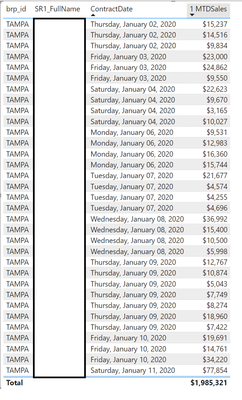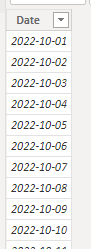cancel
Showing results for
Did you mean:Helper I

## DAX Measure Showing All Records

I am trying to create a MTD measure by fiscal year so we can show sales by store and sales rep. My measure is 100% accurate for the total sales, but when I create a table with the date, store, and sales rep it lists all records, not just the ones from November 2022. All these fields are in one table so I am kind of confused why this is happening.

This is the measure (The MAX is added so it can be dynamic based on a date slider showing any month you want)

1 MTDSales = SUMX(FILTER(JobsWithMilestones, AND(JobsWithMilestones[ContractfiscalYear] = YEAR(MAX(JobsWithMilestones[ContractDate])),JobsWithMilestones[ContractfiscalMonthOfYear] = MONTH(MAX(JobsWithMilestones[ContractDate])))), JobsWithMilestones[GrossAmount])1 ACCEPTED SOLUTIONCommunity Support

Hi @SBIM ,

Because the date fields that you add to the table visual is consecutive,.

For more details, you can read related document: TOTALMTD function (DAX) - DAX | Microsoft Learn , Total Month to Date(TOTALMTD) DAX function in Power Bi (powerbidocs.com)TOTALMTD – DAX Guide

If you only want to show current month, please try below steps:

1. below is my test table

Table:Date Table:

create with dax formula:

``Date Table = CALENDAR(FIRSTDATE('Table'[Date]),LASTDATE('Table'[Date]))``2. create measure with below dax formula

``Total MTD = TOTALMTD(SUM('Table'[Sale]),'Date Table'[Date])``
``````Filter Month =
VAR cur_month =
MONTH ( TODAY () )
VAR _m =
MONTH ( SELECTEDVALUE ( 'Date Table'[Date] ) )
RETURN
IF ( cur_month = _m, 1 )
``````

3. add a table visual with fields and measure, add "Filter Month" to this visual as a filterPlease refer the attached .pbix file.

Best regards,
Community Support Team_ Binbin Yu
If this post helps, then please consider Accept it as the solution to help the other members find it more quickly.

2 REPLIES 2Community Support

Hi @SBIM ,

Because the date fields that you add to the table visual is consecutive,.

For more details, you can read related document: TOTALMTD function (DAX) - DAX | Microsoft Learn , Total Month to Date(TOTALMTD) DAX function in Power Bi (powerbidocs.com)TOTALMTD – DAX Guide

If you only want to show current month, please try below steps:

1. below is my test table

Table:Date Table:

create with dax formula:

``Date Table = CALENDAR(FIRSTDATE('Table'[Date]),LASTDATE('Table'[Date]))``2. create measure with below dax formula

``Total MTD = TOTALMTD(SUM('Table'[Sale]),'Date Table'[Date])``
``````Filter Month =
VAR cur_month =
MONTH ( TODAY () )
VAR _m =
MONTH ( SELECTEDVALUE ( 'Date Table'[Date] ) )
RETURN
IF ( cur_month = _m, 1 )
``````

3. add a table visual with fields and measure, add "Filter Month" to this visual as a filterPlease refer the attached .pbix file.

Best regards,
Community Support Team_ Binbin Yu
If this post helps, then please consider Accept it as the solution to help the other members find it more quickly.Frequent Visitor

The ContractDate it's in the format of an valid date type? (Such as "DD/MM/YYYY" for example)

If it is, you can only bring the month (or day and month if you want) to the table, so you could filter the month easily (there's a possibility of create an current month filter also)